# Texas Go Math Grade 2 Lesson 17.1 Answer Key Measure with a Centimeter Ruler

Refer to our Texas Go Math Grade 2 Answer Key Pdf to score good marks in the exams. Test yourself by practicing the problems from Texas Go Math Grade 2 Lesson 17.1 Answer Key Measure with a Centimeter Ruler.

## Texas Go Math Grade 2 Lesson 17.1 Answer Key Measure with a Centimeter Ruler

Essential Question
How do you use a centimeter ruler to measure lengths?
A ruler can be defined as a tool or device used to measure length and draw straight lines. A ruler or measuring tape can be used to measure lengths in both metric and customary units. Here, the ruler is marked in centimeters (cms) along the top and in inches along the bottom.

Explore
Use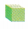to measure the length.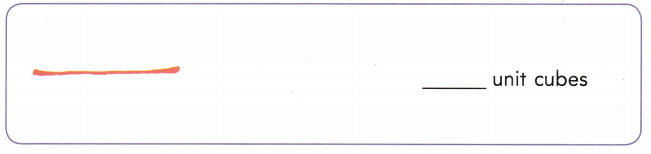Explanation:
measured the length with the help of cubes
The above string measured 6 unit cubes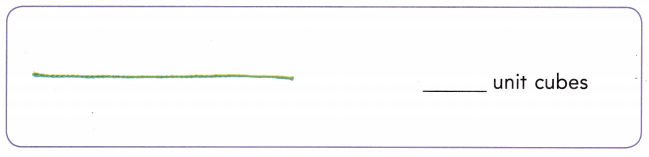Explanation:
measured the length with the help of cubes
The above string measured 11 unit cubes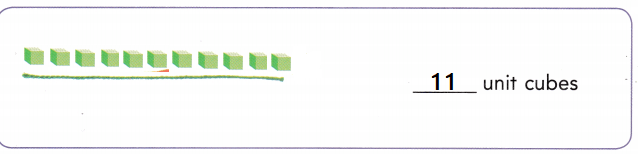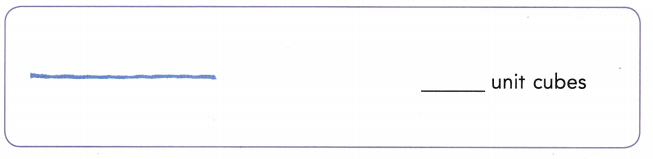Explanation:
measured the length with the help of cubes
The above string measured 8 unit cubes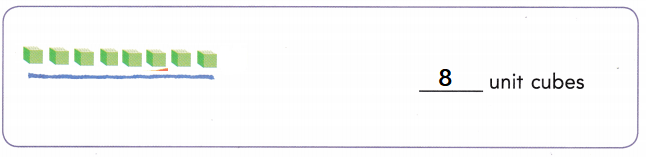Math Talk
Mathematical Processes
Describe how to use unit cubes to measure objects length
Explanation:
Place the cubes on the sting
Then count the cubes

For The Teacher

• Provide unit cubes for children. Remind them that to measure with cubes they should line them up so that there are no gaps.

Model and Draw
What is the length of the crayon to the nearest centimeter?

Line up the left edge of the object with the zero mark on the ruler._______ centimeters
Explanation:
The above crayon is measured with the help of  a ruler
placed the left edge of the object with the zero mark on the ruler.

Share and Show

Measure the length to the nearest centimeter.

Question 1._______ centimetersExplanation:
The above brush is measured with the help of  a ruler
placed the left edge of the object with the zero mark on the ruler.

Question 2.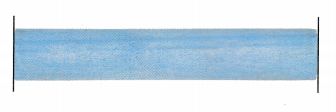_______ centimetersExplanation:
The above pipe is measured with the help of  a ruler
placed the left edge of the object with the zero mark on the ruler.

Question 3.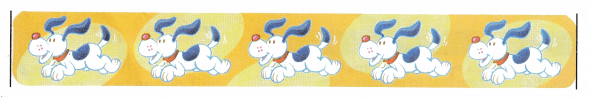_______ centimetersExplanation:
The above scale is measured with the help of  a ruler
placed the left edge of the object with the zero mark on the ruler.

Problem Solving

Measure the length to the nearest centimeter.

Question 4._______ centimetersExplanation:
it measures 13 centimeters
The above brush is measured with the help of  a ruler
placed the left edge of the object with the zero mark on the ruler.

Question 5.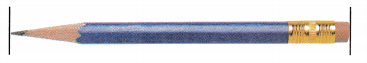_____ centimetersExplanation:
the pencil measures 10 centimeters
The above pencil is measured with the help of  a ruler
placed the left edge of the object with the zero mark on the ruler.

Question 6.
H.O.T. Multi-Step The crayon was on the table next to the centimeter ruler. The left edge of the crayon was not lined up with the zero mark on the ruler.What is the length of the crayon?
Explanation:
The above crayon is measured with the help of  a ruler
placed the left edge of the crayon at 3 was not lined up with the zero mark on the ruler
so, start counting at 4 as 1 then the crayon measures as 8

Question 7.
H.O.T. A marker is 13 centimeters long. This length ends between which two centimeter-marks on a ruler?
Explanation:
placed the left edge of the object with the zero mark on the ruler.
The end will be at 13

Question 8.
Use Tool Measure with a centimeter ruler. How many centimeters long is the pencil?(A) 10 centimeters
(B) 13 centimeters
(C) 11 centimeters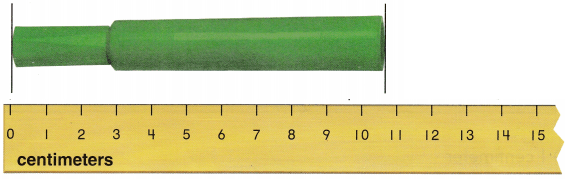Explanation:
11 centimeters long
The above crayon is measured with the help of  a ruler
placed the left edge of the object with the zero mark on the ruler.

Question 9.
Use Tools Measure with a centimeter ruler. How many centimeters long is the marker?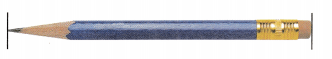(A) 9 centimeters
(B) 12 centimeters
(C) 10 centimeters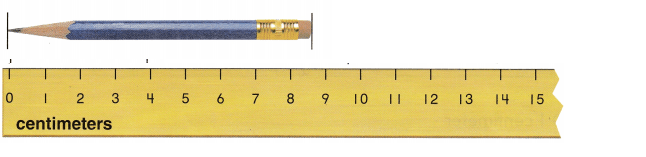Explanation:
it measures 9 centimeters
The above pencil  is measured with the help of  a ruler
placed the left edge of the object with the zero mark on the ruler.

Question 10.
Texas Test Prep Use a centimeter ruler. Which is the best choice for the length of this string?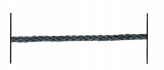(A) 9 centimeters
(B) 12 centimeters
(C) 10 centimetersExplanation:
9 is the best choice for it
The above string is measured with the help of  a ruler
placed the left edge of the object with the zero mark on the ruler.

### Texas Go Math Grade 2 Lesson 17.1 Homework and Practice Answer Key

Measure the length to the nearest centimeter.

Question 1.___ centimeters
Explanation:
The above string is measured with the help of  a ruler
placed the left edge of the object with the zero mark on the ruler.
it measures 10 centimeters

Problem Solving

Question 2.
Multi-Step A pencil was on a table next to a centimeter ruler. The left edge of the pencil was not lined up with the zero mark on the ruler.What is the length of the pencil? Explain how you found your answer.
Explanation:
The above pencil is measured with the help of  a ruler
placed the left edge of the crayon at 1 and started counting from 2
13 – 1 = 12

Texas Test Prep

Lesson Check

Question 3.
How many centimeters long is the rope?(A) 1 centimeters
(B) 8 centimeter
(C) 3 centimeters
Explanation:
The above rope is measured with the help of  a ruler
placed the left edge of the object with the zero mark on the ruler.
It measures 8 centimeters

Question 4.
Melanie has a paintbrush that is shown. How many centimeters long is her paintbrush?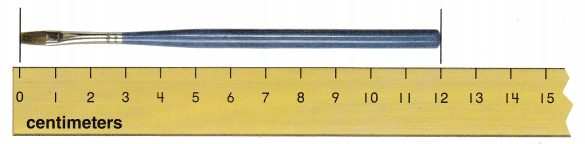(A) 18 centimeters
(B) 5 centimeters
(C) 12 centimeters